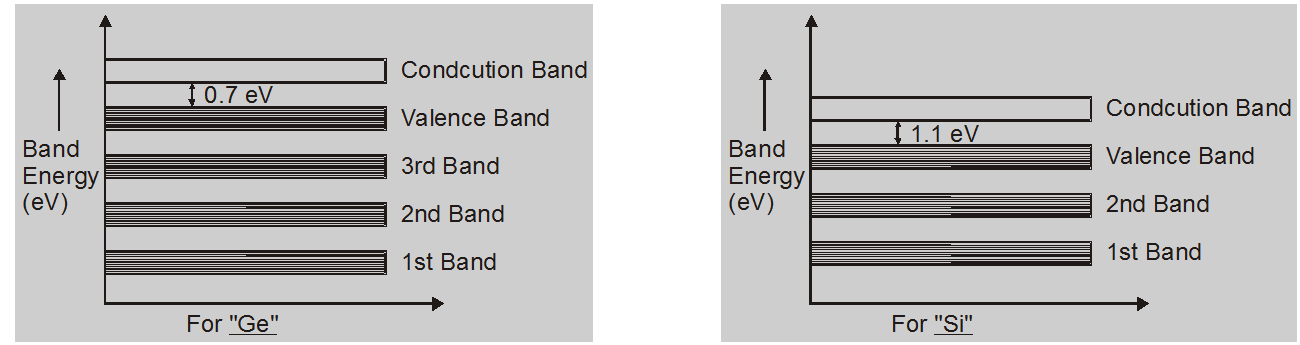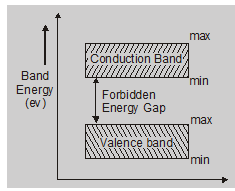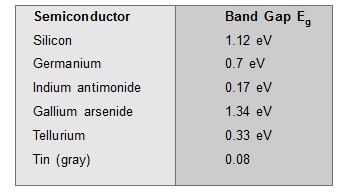# Energy band theory in solids - Electronics, Physics - eSaral

Hey, do you want to learn about the Energy band theory in solids? If yes. Then you are at the right place.

## Energy Bands in solid### Valence band:

The electrons in the outermost shells are not strongly bonded to their nuclei, these electrons are valence electrons. The band formed by these electrons is called the valence band.

### Conduction band:

Valence electrons are loosely attached to the nucleus. Even at ordinary temperature, some of the valence electrons left the valence band. These are called free electrons and also called conduction electrons. The band occupied by these electrons is called the conduction band.

### Forbidden energy gap ($\Delta$Eg.):The separation between the conduction band and valence band is known as the forbidden energy gap.

$\Delta \mathrm{Eg} .=(\mathrm{CB})_{\mathrm{Min}}-(\mathrm{VB})_{\mathrm{Max}}$

• No free electrons are in F.E.G.

• The width of FEG depends upon the nature of the substance

• Width is more than valence electrons are strongly attached with the nucleus

• The width of FEG represent in eV

• If the conduction band is empty, then-current conduction is not possible.

• The Greater is the energy gap, the more tightly the valence electrons are bounded to the nucleus. The energy gap in some semiconductors is as follows-The energy gap decreases slightly with an increase in temperature.